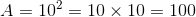# SSAT Middle Level Math : How to find a square on a coordinate plane

## Example Questions

### Example Question #1 : How To Find A Square On A Coordinate Plane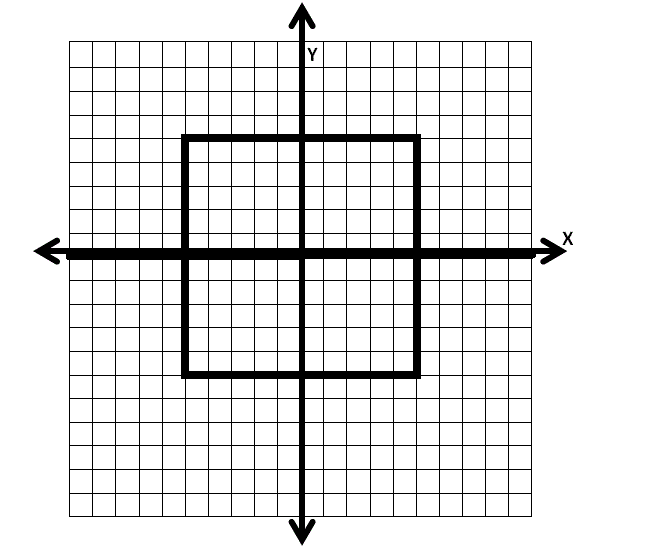The above square has an area ofsquare units. What fraction of the area of square is in quadrant II?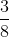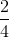Explanation:

To find the fraction of the entire squares area that lays in quadrant II, notice that the square is taking up an equivalent amount in each of the four quadrants. Thus,of the squares area is in quadrant II.

Also note that the area of the square that lays in quadrant II issquare units, thus the problem could have alternatively been solved by reducing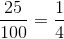.

### Example Question #2 : How To Find A Square On A Coordinate Plane

If a square has an area ofsquare units, what is the perimeter?Explanation:

In order to solve this problem, apply the formula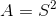, in order to conclude that the length of side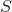must equalfor the area to equalOnce you've found the length of side, apply the formula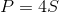.

Thus, the solution is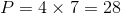### Example Question #3 : How To Find A Square On A Coordinate Plane

A square has an area ofsquare units, what is the perimeter?Explanation:

In order to solve this problem, apply the formula, in order to conclude that the length of sidemust equalfor the area to equalOnce you've found the length of side, apply the formula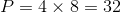### Example Question #4 : How To Find A Square On A Coordinate Plane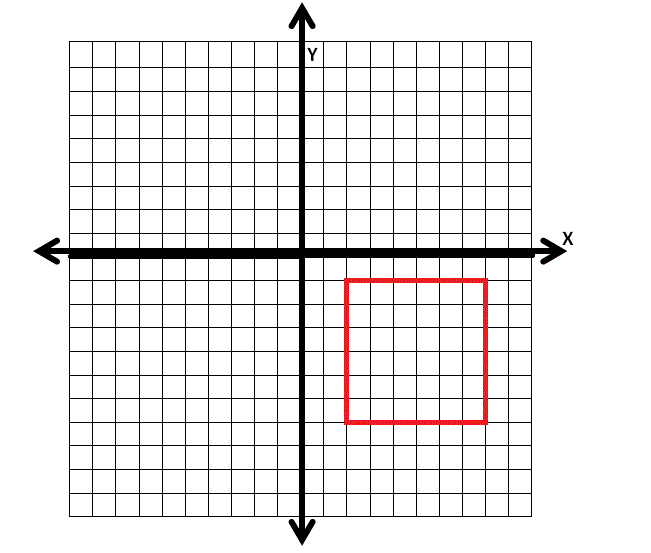Find the perimeter of the square shown above.Explanation:

To find the perimeter of this square, apply the formula:, where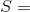the length of one side of the square.

Thus, the solution is: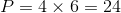### Example Question #5 : How To Find A Square On A Coordinate Plane

A square has an area of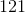square units, what is the perimeter of the square?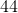Explanation:

In order to solve this problem, apply the formula, in order to conclude that the length of sidemust equalfor the area to equalOnce you've found the length of side, apply the formula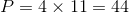### Example Question #32 : Coordinate Geometry

A square has an area ofsquare units. Find the perimeter.Explanation:

In order to solve this problem, apply the formula, in order to conclude that the length of sidemust equalfor the area to equalOnce you've found the length of side, apply the formula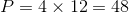### Example Question #33 : Coordinate GeometryFind the area of the above square.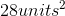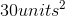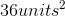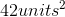Explanation:

In order to find the area of the square above apply the formula:, wherethe length of one side of the square.

Since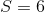the solution is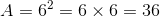### Example Question #34 : Coordinate GeometryFind the area of the square shown above.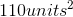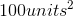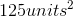Explanation:

In order to find the area of the above square, apply the formula:, whenequals the length of one side of the square.

Since,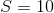the solution is: